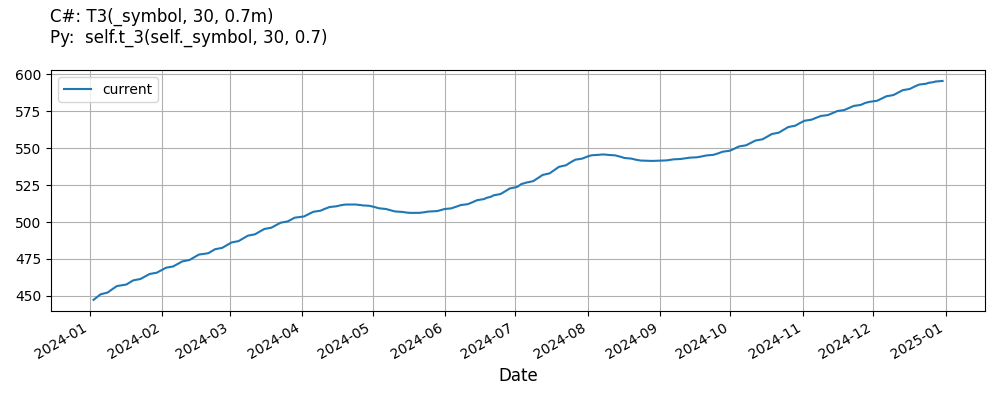# Supported Indicators

## T3 Moving Average

### Introduction

This indicator computes the T3 Moving Average (T3). The T3 Moving Average is calculated with the following formula: EMA1(x, Period) = EMA(x, Period) EMA2(x, Period) = EMA(EMA1(x, Period),Period) GD(x, Period, volumeFactor) = (EMA1(x, Period)*(1+volumeFactor)) - (EMA2(x, Period)* volumeFactor) T3 = GD(GD(GD(t, Period, volumeFactor), Period, volumeFactor), Period, volumeFactor);

To view the implementation of this indicator, see the LEAN GitHub repository.

### Using T3 Indicator

To create an automatic indicators for T3MovingAverage, call the T3 helper method from the QCAlgorithm class. The T3 method creates a T3MovingAverage object, hooks it up for automatic updates, and returns it so you can used it in your algorithm. In most cases, you should call the helper method in the Initialize method.

public class T3MovingAverageAlgorithm : QCAlgorithm
{
private Symbol _symbol;
private T3MovingAverage _t3;

public override void Initialize()
{
_t3 = T3(_symbol, 30, 0.7);
}

public override void OnData(Slice data)
{
{
// The current value of _t3 is represented by itself (_t3)
// or _t3.Current.Value
Plot("T3MovingAverage", "t3", _t3);

}
}
}
class T3MovingAverageAlgorithm(QCAlgorithm):
def Initialize(self) -> None:
self.t3 = self.T3(self.symbol, 30, 0.7)

def OnData(self, slice: Slice) -> None:
# The current value of self.t3 is represented by self.t3.Current.Value
self.Plot("T3MovingAverage", "t3", self.t3.Current.Value)



The following reference table describes the T3 method:

### T3()1/1

            T3MovingAverage QuantConnect.Algorithm.QCAlgorithm.T3 (
Symbol                           symbol,
Int32                            period,
*Decimal                         volumeFactor,
*Nullable<Resolution>      resolution,
*Func<IBaseData, Decimal>  selector
)


Creates a new T3MovingAverage indicator.

If you don't provide a resolution, it defaults to the security resolution. If you provide a resolution, it must be greater than or equal to the resolution of the security. For instance, if you subscribe to hourly data for a security, you should update its indicator with data that spans 1 hour or longer.

You can manually create a T3MovingAverage indicator, so it doesn't automatically update. Manual indicators let you update their values with any data you choose.

Updating your indicator manually enables you to control when the indicator is updated and what data you use to update it. To manually update the indicator, call the Update method with time/number pair, or an IndicatorDataPoint. The indicator will only be ready after you prime it with enough data.

public class T3MovingAverageAlgorithm : QCAlgorithm
{
private Symbol _symbol;
private T3MovingAverage _t3;

public override void Initialize()
{
_t3 = new T3MovingAverage(30, 0.7);
}

public override void OnData(Slice data)
{
if (data.Bars.TryGeValue(_symbol, out var bar))
{
_t3.Update(bar.EndTime, bar.Close);
}

{
// The current value of _t3 is represented by itself (_t3)
// or _t3.Current.Value
Plot("T3MovingAverage", "t3", _t3);

}
}
}
class T3MovingAverageAlgorithm(QCAlgorithm):
def Initialize(self) -> None:
self.t3 = T3MovingAverage(30, 0.7)

def OnData(self, slice: Slice) -> None:
bar = slice.Bars.get(self.symbol)
if bar:
self.t3.Update(bar.EndTime, bar.Close)

# The current value of self.t3 is represented by self.t3.Current.Value
self.Plot("T3MovingAverage", "t3", self.t3.Current.Value)



To register a manual indicator for automatic updates with the security data, call the RegisterIndicator method.

public class T3MovingAverageAlgorithm : QCAlgorithm
{
private Symbol _symbol;
private T3MovingAverage _t3;

public override void Initialize()
{
_t3 = new T3MovingAverage(30, 0.7);
RegisterIndicator(_symbol, _t3, Resolution.Daily);
}

public override void OnData(Slice data)
{
{
// The current value of _t3 is represented by itself (_t3)
// or _t3.Current.Value
Plot("T3MovingAverage", "t3", _t3);

}
}
}
class T3MovingAverageAlgorithm(QCAlgorithm):
def Initialize(self) -> None:
self.t3 = T3MovingAverage(30, 0.7)
self.RegisterIndicator(self.symbol, self.t3, Resolution.Daily)

def OnData(self, slice: Slice) -> None:
# The current value of self.t3 is represented by self.t3.Current.Value
self.Plot("T3MovingAverage", "t3", self.t3.Current.Value)



The following reference table describes the T3MovingAverage constructor:

### T3MovingAverage()1/2

            T3MovingAverage QuantConnect.Indicators.T3MovingAverage (
string    name,
int       period,
*decimal  volumeFactor
)


Initializes a new instance of the T3MovingAverage class using the specified name and period.

### T3MovingAverage()2/2

            T3MovingAverage QuantConnect.Indicators.T3MovingAverage (
int       period,
*decimal  volumeFactor
)


Initializes a new instance of the T3MovingAverage class using the specified period.

### Visualization

The following image shows plot values of selected properties of T3MovingAverage using the plotly library.You can also see our Videos. You can also get in touch with us via Discord.